# kinematicTrajectory

Rate-driven trajectory generator

## Description

The `kinematicTrajectory` System object™ generates trajectories using specified acceleration and angular velocity.

To generate a trajectory from rates:

1. Create the `kinematicTrajectory` object and set its properties.

2. Call the object with arguments, as if it were a function.

## Creation

### Syntax

``trajectory = kinematicTrajectory``
``trajectory = kinematicTrajectory(Name,Value)``

### Description

example

````trajectory = kinematicTrajectory` returns a System object, `trajectory`, that generates a trajectory based on acceleration and angular velocity.```

example

````trajectory = kinematicTrajectory(Name,Value)` sets each property `Name` to the specified `Value`. Unspecified properties have default values.Example: ```trajectory = kinematicTrajectory('SampleRate',200,'Position',[0,1,10])``` creates a kinematic trajectory System object, `trajectory`, with a sample rate of 200 Hz and the initial position set to [0,1,10].```

## Properties

expand all

If a property is tunable, you can change its value at any time.

Sample rate of trajectory in Hz, specified as a positive scalar.

Tunable: Yes

Data Types: `single` | `double`

Position state in the local navigation coordinate system in meters, specified as a three-element row vector.

Tunable: Yes

Data Types: `single` | `double`

Velocity state in the local navigation coordinate system in m/s, specified as a three-element row vector.

Tunable: Yes

Data Types: `single` | `double`

Orientation state in the local navigation coordinate system, specified as a scalar quaternion or 3-by-3 real matrix. The orientation is a frame rotation from the local navigation coordinate system to the current body frame.

Tunable: Yes

Data Types: `quaternion` | `single` | `double`

Source of acceleration state, specified as `'Input'` or `'Property'`.

• `'Input'` –– specify acceleration state as an input argument to the kinematic trajectory object

• `'Property'` –– specify acceleration state by setting the `Acceleration` property

Tunable: No

Data Types: `char` | `string`

Acceleration state in m/s2, specified as a three-element row vector.

Tunable: Yes

#### Dependencies

To enable this property, set AccelerationSource to `'Property'`.

Data Types: `single` | `double`

Source of angular velocity state, specified as `'Input'` or `'Property'`.

• `'Input'` –– specify angular velocity state as an input argument to the kinematic trajectory object

• `'Property'` –– specify angular velocity state by setting the `AngularVelocity` property

Tunable: No

Data Types: `char` | `string`

Angular velocity state in rad/s, specified as a three-element row vector.

Tunable: Yes

#### Dependencies

To enable this property, set AngularVelocitySource to `'Property'`.

Data Types: `single` | `double`

Number of samples per output frame, specified as a positive integer.

Tunable: No

#### Dependencies

To enable this property, set AngularVelocitySource to `'Property'` and AccelerationSource to `'Property'`.

Data Types: `single` | `double`

## Usage

### Syntax

``[position,orientation,velocity,acceleration,angularVelocity] = trajectory(bodyAcceleration,bodyAngularVelocity)``
``[position,orientation,velocity,acceleration,angularVelocity] = trajectory(bodyAngularVelocity)``
``[position,orientation,velocity,acceleration,angularVelocity] = trajectory(bodyAcceleration)``
``[position,orientation,velocity,acceleration,angularVelocity] = trajectory()``

### Description

example

````[position,orientation,velocity,acceleration,angularVelocity] = trajectory(bodyAcceleration,bodyAngularVelocity)` outputs the trajectory state and then updates the trajectory state based on `bodyAcceleration` and `bodyAngularVelocity`.This syntax is only valid if `AngularVelocitySource` is set to `'Input'` and `AccelerationSource` is set to `'Input'`.```
````[position,orientation,velocity,acceleration,angularVelocity] = trajectory(bodyAngularVelocity)` outputs the trajectory state and then updates the trajectory state based on `bodyAngularAcceleration`.This syntax is only valid if `AngularVelocitySource` is set to `'Input'` and `AccelerationSource` is set to `'Property'`.```
````[position,orientation,velocity,acceleration,angularVelocity] = trajectory(bodyAcceleration)` outputs the trajectory state and then updates the trajectory state based on `bodyAcceleration`.This syntax is only valid if `AngularVelocitySource` is set to `'Property'` and `AccelerationSource` is set to `'Input'`.```
````[position,orientation,velocity,acceleration,angularVelocity] = trajectory()` outputs the trajectory state and then updates the trajectory state.This syntax is only valid if `AngularVelocitySource` is set to `'Property'` and `AccelerationSource` is set to `'Property'`.```

### Input Arguments

expand all

Acceleration in the body coordinate system in meters per second squared, specified as an N-by-3 matrix.

N is the number of samples in the current frame.

Angular velocity in the body coordinate system in radians per second, specified as an N-by-3 matrix.

N is the number of samples in the current frame.

### Output Arguments

expand all

Position in the local navigation coordinate system in meters, returned as an N-by-3 matrix.

N is the number of samples in the current frame.

Data Types: `single` | `double`

Orientation in the local navigation coordinate system, returned as an N-by-1 quaternion column vector or a 3-by-3-by-N real array. Each quaternion or 3-by-3 rotation matrix is a frame rotation from the local navigation coordinate system to the current body coordinate system.

N is the number of samples in the current frame.

Data Types: `single` | `double`

Velocity in the local navigation coordinate system in meters per second, returned as an N-by-3 matrix.

N is the number of samples in the current frame.

Data Types: `single` | `double`

Acceleration in the local navigation coordinate system in meters per second squared, returned as an N-by-3 matrix.

N is the number of samples in the current frame.

Data Types: `single` | `double`

Angular velocity in the local navigation coordinate system in radians per second, returned as an N-by-3 matrix.

N is the number of samples in the current frame.

Data Types: `single` | `double`

## Object Functions

expand all

 `perturbations` Perturbation defined on object `perturb` Apply perturbations to object
 `step` Run System object algorithm

## Examples

collapse all

Create a default `kinematicTrajectory` System object™ and explore the relationship between input, properties, and the generated trajectories.

`trajectory = kinematicTrajectory`
```trajectory = kinematicTrajectory with properties: SampleRate: 100 Position: [0 0 0] Orientation: [1x1 quaternion] Velocity: [0 0 0] AccelerationSource: 'Input' AngularVelocitySource: 'Input' ```

By default, the `kinematicTrajectory` object has an initial position of [0 0 0] and an initial velocity of [0 0 0]. Orientation is described by a quaternion one (1 + 0i + 0j + 0k).

The `kinematicTrajectory` object maintains a visible and writable state in the properties `Position`, `Velocity`, and `Orientation`. When you call the object, the state is output and then updated.

For example, call the object by specifying an acceleration and angular velocity relative to the body coordinate system.

```bodyAcceleration = [5,5,0]; bodyAngularVelocity = [0,0,1]; [position,orientation,velocity,acceleration,angularVelocity] = trajectory(bodyAcceleration,bodyAngularVelocity)```
```position = 1×3 0 0 0 ```
```orientation = quaternion 1 + 0i + 0j + 0k ```
```velocity = 1×3 0 0 0 ```
```acceleration = 1×3 5 5 0 ```
```angularVelocity = 1×3 0 0 1 ```

The position, orientation, and velocity output from the `trajectory` object correspond to the state reported by the properties before calling the object. The `trajectory` state is updated after being called and is observable from the properties:

`trajectory`
```trajectory = kinematicTrajectory with properties: SampleRate: 100 Position: [2.5000e-04 2.5000e-04 0] Orientation: [1x1 quaternion] Velocity: [0.0500 0.0500 0] AccelerationSource: 'Input' AngularVelocitySource: 'Input' ```

The `acceleration` and `angularVelocity` output from the `trajectory` object correspond to the `bodyAcceleration` and `bodyAngularVelocity`, except that they are returned in the navigation coordinate system. Use the `orientation` output to rotate `acceleration` and `angularVelocity` to the body coordinate system and verify they are approximately equivalent to `bodyAcceleration` and `bodyAngularVelocity`.

`rotatedAcceleration = rotatepoint(orientation,acceleration)`
```rotatedAcceleration = 1×3 5 5 0 ```
`rotatedAngularVelocity = rotatepoint(orientation,angularVelocity)`
```rotatedAngularVelocity = 1×3 0 0 1 ```

The `kinematicTrajectory` System object™ enables you to modify the trajectory state through the properties. Set the position to [0,0,0] and then call the object with a specified acceleration and angular velocity in the body coordinate system. For illustrative purposes, clone the `trajectory` object before modifying the `Position` property. Call both objects and observe that the positions diverge.

```trajectoryClone = clone(trajectory); trajectory.Position = [0,0,0]; position = trajectory(bodyAcceleration,bodyAngularVelocity)```
```position = 1×3 0 0 0 ```
`clonePosition = trajectoryClone(bodyAcceleration,bodyAngularVelocity)`
```clonePosition = 1×3 10-3 × 0.2500 0.2500 0 ```

This example shows how to create a trajectory oscillating along the North axis of a local NED coordinate system using the `kinematicTrajectory` System object™.

Create a default `kinematicTrajectory` object. The default initial orientation is aligned with the local NED coordinate system.

```traj = kinematicTrajectory ```
```traj = kinematicTrajectory with properties: SampleRate: 100 Position: [0 0 0] Orientation: [1x1 quaternion] Velocity: [0 0 0] AccelerationSource: 'Input' AngularVelocitySource: 'Input' ```

Define a trajectory for a duration of 10 seconds consisting of rotation around the East axis (pitch) and an oscillation along North axis of the local NED coordinate system. Use the default `kinematicTrajectory` sample rate.

```fs = traj.SampleRate; duration = 10; numSamples = duration*fs; cyclesPerSecond = 1; samplesPerCycle = fs/cyclesPerSecond; numCycles = ceil(numSamples/samplesPerCycle); maxAccel = 20; triangle = [linspace(maxAccel,1/fs-maxAccel,samplesPerCycle/2), ... linspace(-maxAccel,maxAccel-(1/fs),samplesPerCycle/2)]'; oscillation = repmat(triangle,numCycles,1); oscillation = oscillation(1:numSamples); accNED = [zeros(numSamples,2),oscillation]; angVelNED = zeros(numSamples,3); angVelNED(:,2) = 2*pi; ```

Plot the acceleration control signal.

```timeVector = 0:1/fs:(duration-1/fs); figure(1) plot(timeVector,oscillation) xlabel('Time (s)') ylabel('Acceleration (m/s)^2') title('Acceleration in Local NED Coordinate System') ```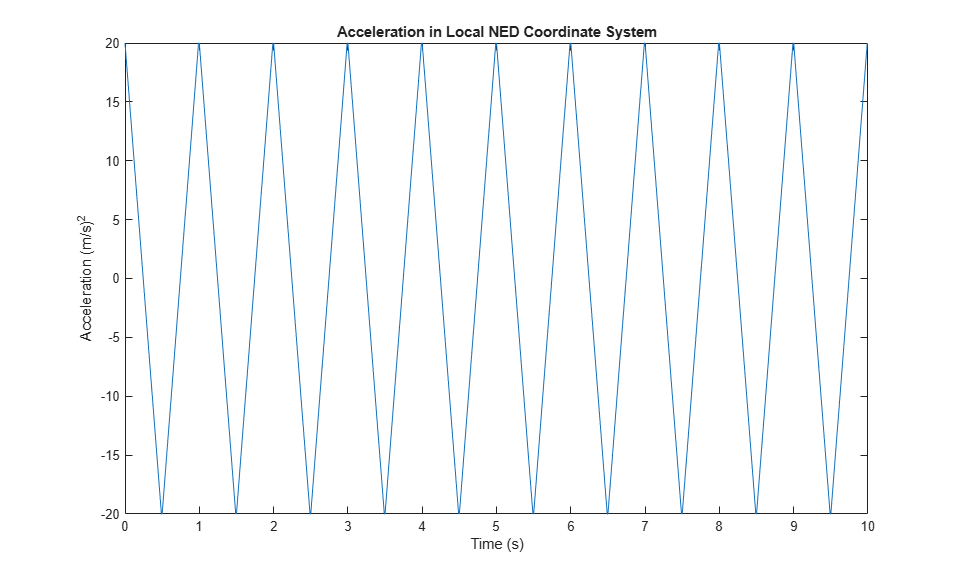Generate the trajectory sample-by-sample in a loop. The `kinematicTrajectory` System object assumes the acceleration and angular velocity inputs are in the local sensor body coordinate system. Rotate the acceleration and angular velocity control signals from the NED coordinate system to the sensor body coordinate system using `rotateframe` and the `Orientation` state. Update a 3-D plot of the position at each time. Add `pause` to mimic real-time processing. Once the loop is complete, plot the position over time. Rotating the `accNED` and `angVelNED` control signals to the local body coordinate system assures the motion stays along the Down axis.

```figure(2) plotHandle = plot3(traj.Position(1),traj.Position(2),traj.Position(3),'bo'); grid on xlabel('North') ylabel('East') zlabel('Down') axis([-1 1 -1 1 0 1.5]) hold on q = ones(numSamples,1,'quaternion'); for ii = 1:numSamples accBody = rotateframe(traj.Orientation,accNED(ii,:)); angVelBody = rotateframe(traj.Orientation,angVelNED(ii,:)); [pos(ii,:),q(ii),vel,ac] = traj(accBody,angVelBody); set(plotHandle,'XData',pos(ii,1),'YData',pos(ii,2),'ZData',pos(ii,3)) pause(1/fs) end figure(3) plot(timeVector,pos(:,1),'bo',... timeVector,pos(:,2),'r.',... timeVector,pos(:,3),'g.') xlabel('Time (s)') ylabel('Position (m)') title('NED Position Over Time') legend('North','East','Down') ```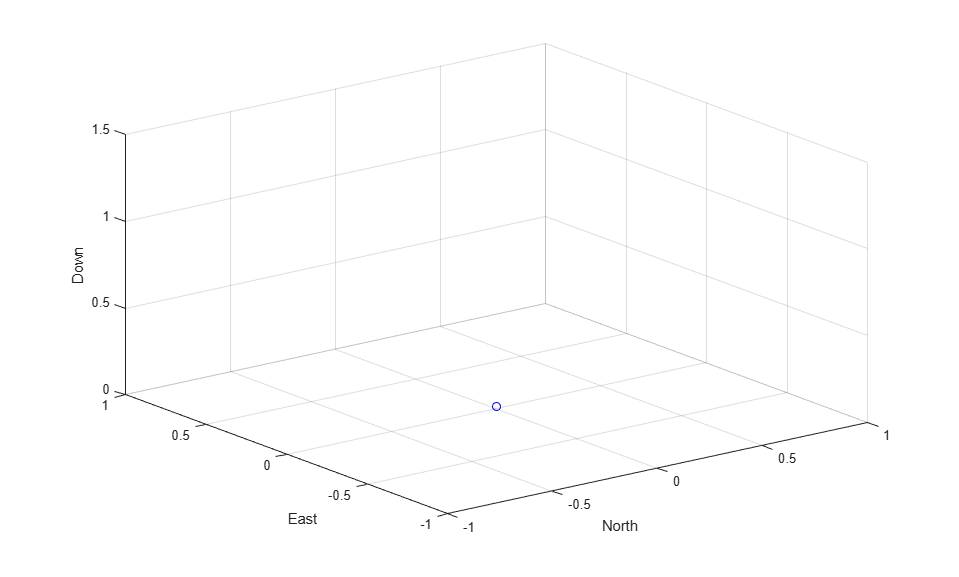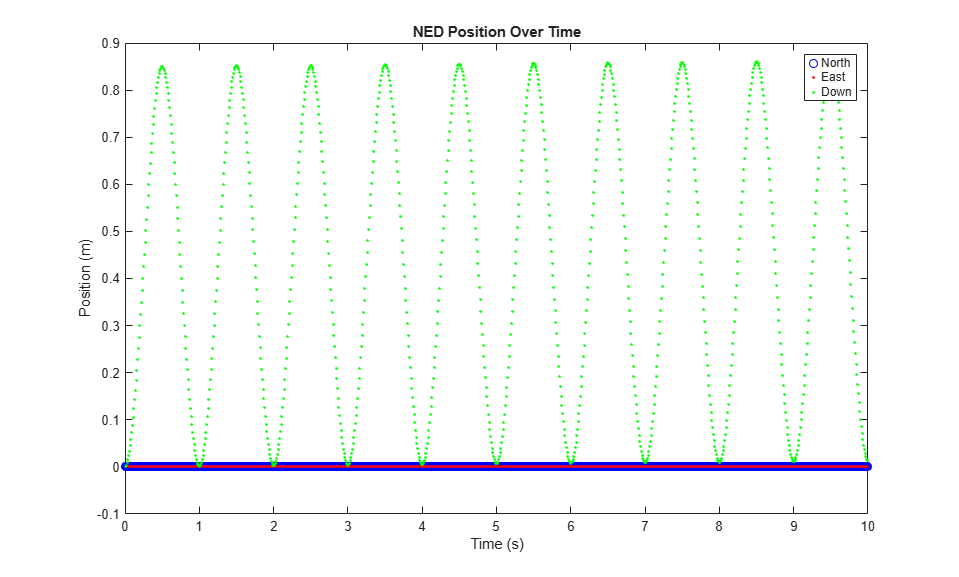Convert the recorded orientation to Euler angles and plot. Although the orientation of the platform changed over time, the acceleration always acted along the North axis.

```figure(4) eulerAngles = eulerd(q,'ZYX','frame'); plot(timeVector,eulerAngles(:,1),'bo',... timeVector,eulerAngles(:,2),'r.',... timeVector,eulerAngles(:,3),'g.') axis([0,duration,-180,180]) legend('Yaw','Pitch','Roll') xlabel('Time (s)') ylabel('Rotation (degrees)') title('Orientation') ```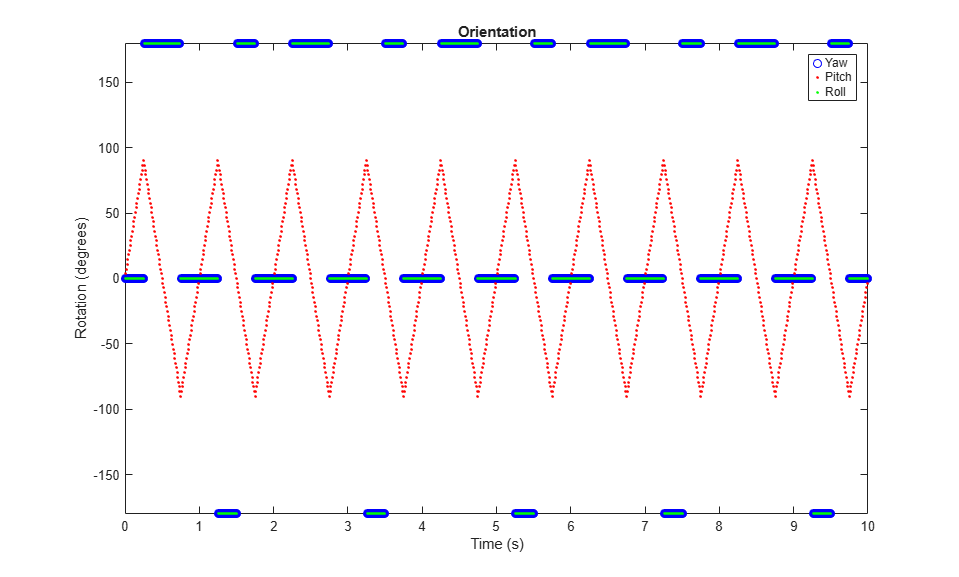This example shows how to generate a coil trajectory using the `kinematicTrajectory` System object™.

Create a circular trajectory for a 1000 second duration and a sample rate of 10 Hz. Set the radius of the circle to 5000 meters and the speed to 80 meters per second. Set the climb rate to 100 meters per second and the pitch to 15 degrees. Specify the initial orientation as pointed in the direction of motion.

```duration = 1000; % seconds fs = 10; % Hz N = duration*fs; % number of samples radius = 5000; % meters speed = 80; % meters per second climbRate = 50; % meters per second initialYaw = 90; % degrees pitch = 15; % degrees initPos = [radius, 0, 0]; initVel = [0, speed, climbRate]; initOrientation = quaternion([initialYaw,pitch,0],'eulerd','zyx','frame'); trajectory = kinematicTrajectory('SampleRate',fs, ... 'Velocity',initVel, ... 'Position',initPos, ... 'Orientation',initOrientation);```

Specify a constant acceleration and angular velocity in the body coordinate system. Rotate the body frame to account for the pitch.

```accBody = zeros(N,3); accBody(:,2) = speed^2/radius; accBody(:,3) = 0.2; angVelBody = zeros(N,3); angVelBody(:,3) = speed/radius; pitchRotation = quaternion([0,pitch,0],'eulerd','zyx','frame'); angVelBody = rotateframe(pitchRotation,angVelBody); accBody = rotateframe(pitchRotation,accBody);```

Call `trajectory` with the specified acceleration and angular velocity in the body coordinate system. Plot the position, orientation, and speed over time.

```[position, orientation, velocity] = trajectory(accBody,angVelBody); eulerAngles = eulerd(orientation,'ZYX','frame'); speed = sqrt(sum(velocity.^2,2)); timeVector = (0:(N-1))/fs; figure(1) plot3(position(:,1),position(:,2),position(:,3)) xlabel('North (m)') ylabel('East (m)') zlabel('Down (m)') title('Position') grid on```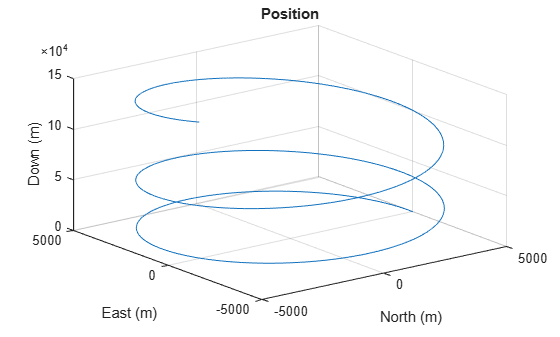```figure(2) plot(timeVector,eulerAngles(:,1),... timeVector,eulerAngles(:,2),... timeVector,eulerAngles(:,3)) axis([0,duration,-180,180]) legend('Yaw (Rotation Around Down)','Pitch (Rotation Around East)','Roll (Rotation Around North)') xlabel('Time (s)') ylabel('Rotation (degrees)') title('Orientation')```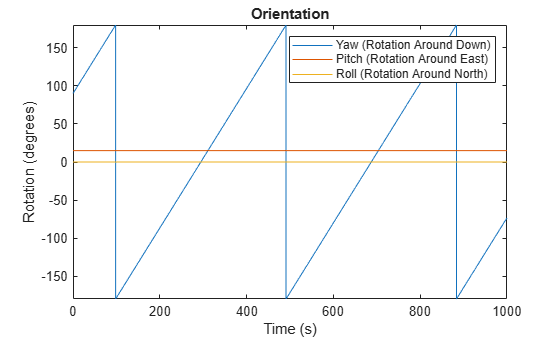```figure(3) plot(timeVector,speed) xlabel('Time (s)') ylabel('Speed (m/s)') title('Speed')```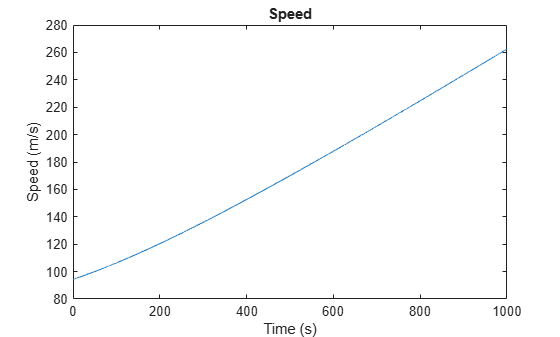Define a constant angular velocity and constant acceleration that describe a spiraling circular trajectory.

```Fs = 100; r = 10; speed = 2.5; initialYaw = 90; initPos = [r 0 0]; initVel = [0 speed 0]; initOrient = quaternion([initialYaw 0 0], 'eulerd', 'ZYX', 'frame'); accBody = [0 speed^2/r 0.01]; angVelBody = [0 0 speed/r];```

Create a kinematic trajectory object.

```traj = kinematicTrajectory('SampleRate',Fs, ... 'Position',initPos, ... 'Velocity',initVel, ... 'Orientation',initOrient, ... 'AccelerationSource','Property', ... 'Acceleration',accBody, ... 'AngularVelocitySource','Property', ... 'AngularVelocity',angVelBody);```

Call the kinematic trajectory object in a loop and log the position output. Plot the position over time.

```N = 10000; pos = zeros(N, 3); for i = 1:N pos(i,:) = traj(); end plot3(pos(:,1), pos(:,2), pos(:,3)) title('Position') xlabel('X (m)') ylabel('Y (m)') zlabel('Z (m)')```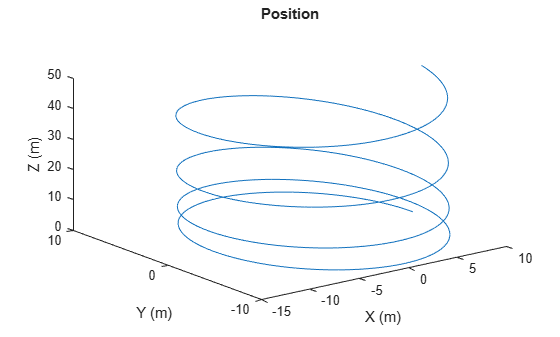## Version History

Introduced in R2018b